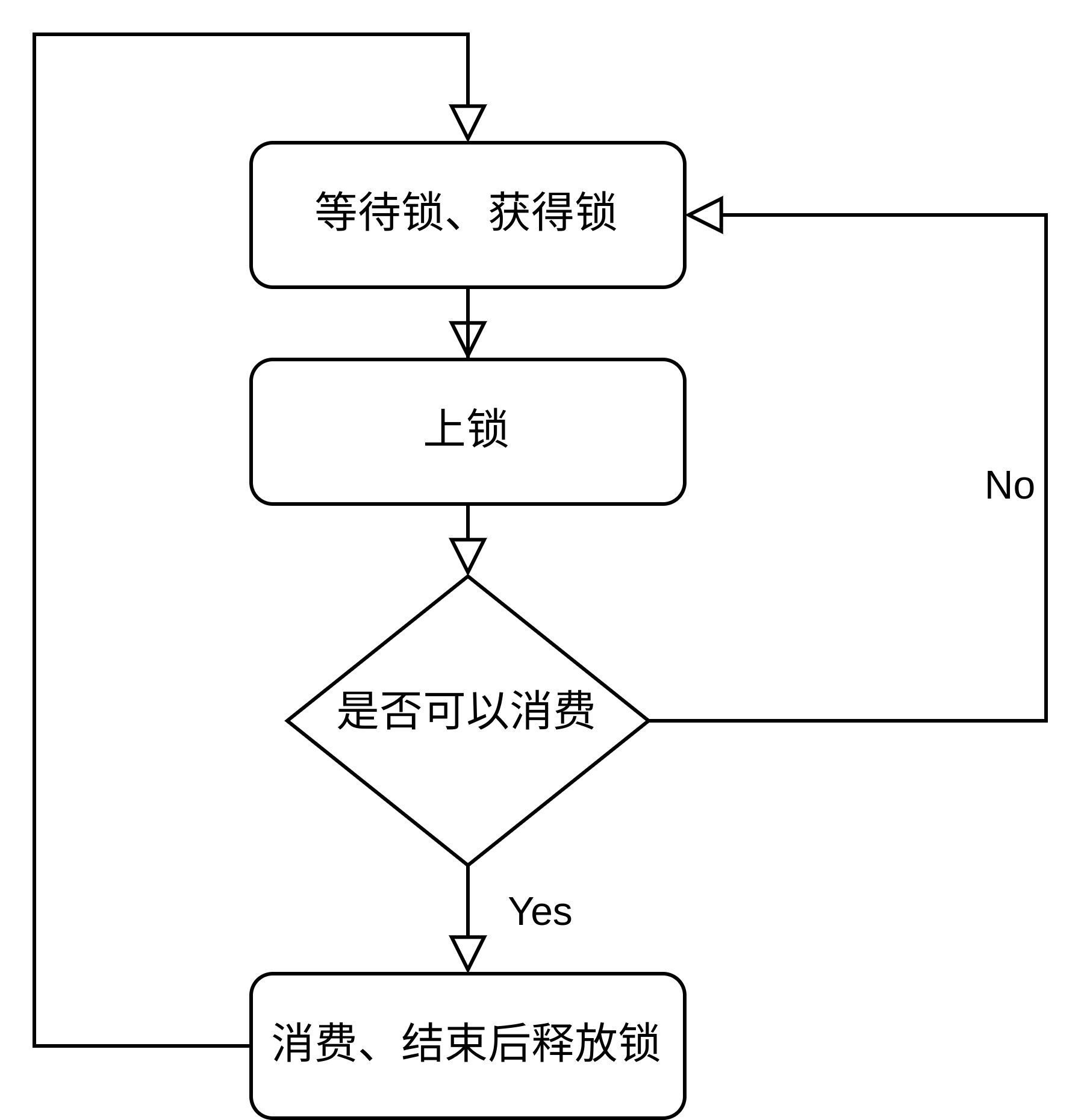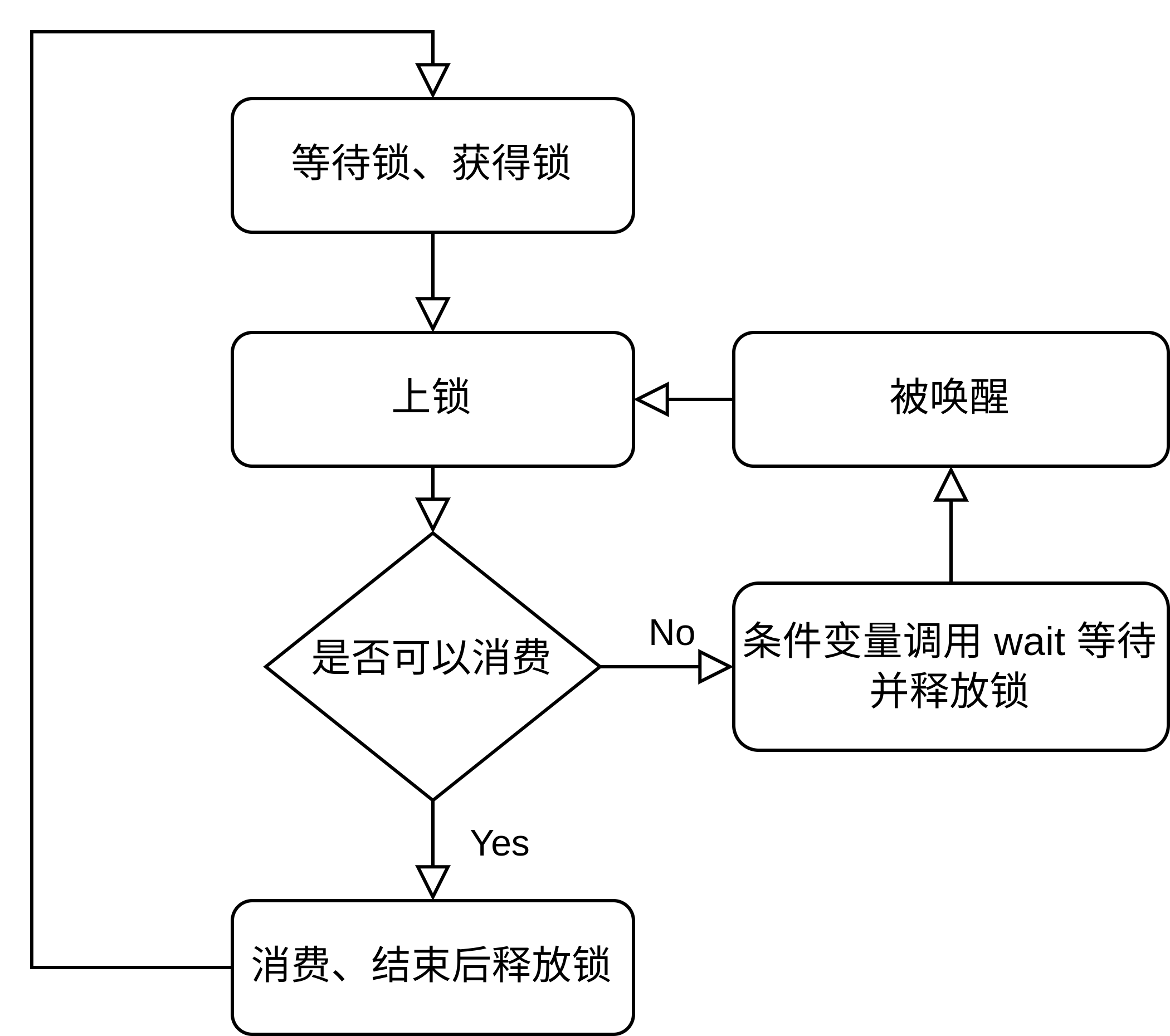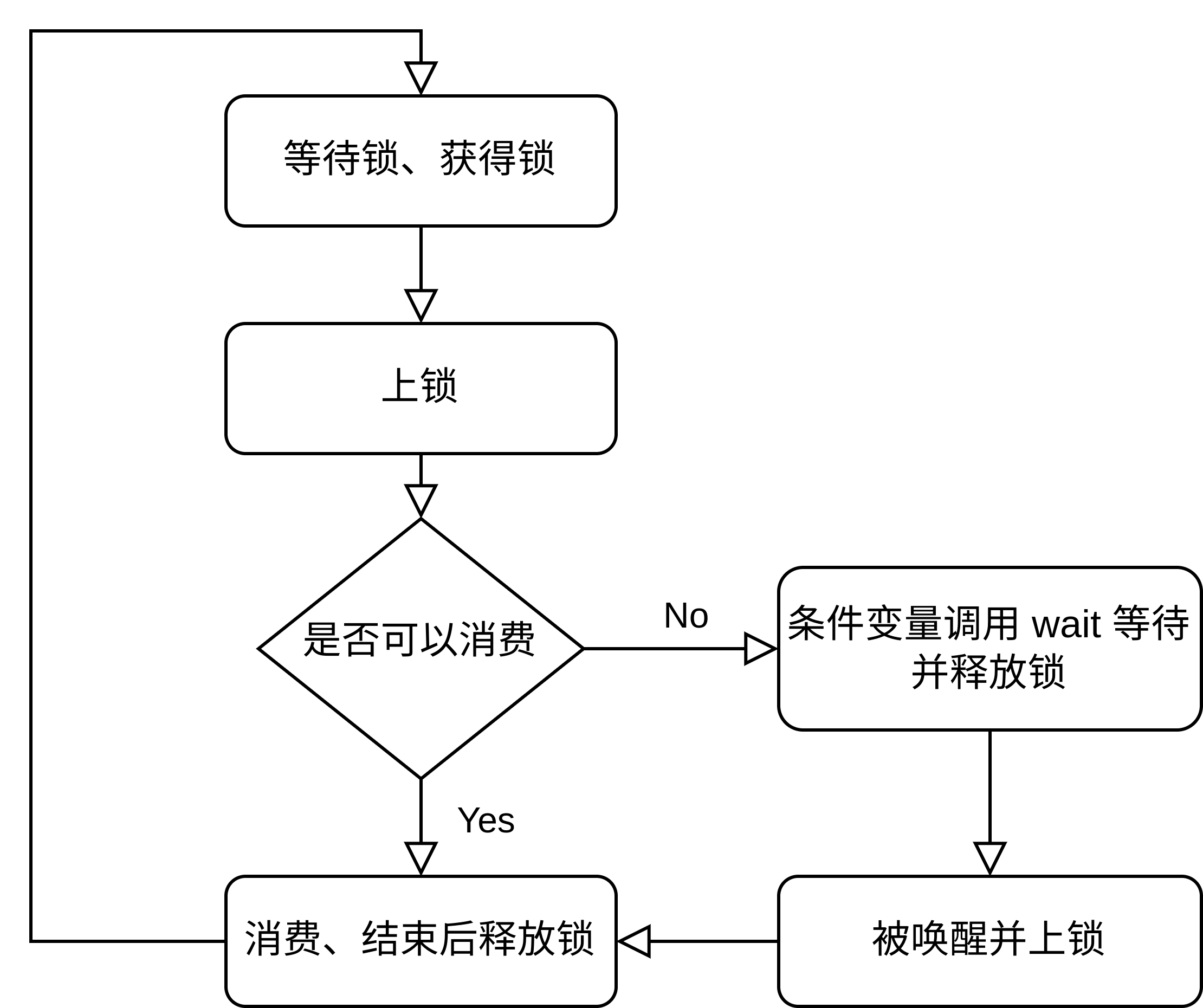# 为什么要使用条件变量？#

## 没有条件变量我们如何实现相同的需求？#

### 不使用条件变量版本#

``````#include <queue>
#include <chrono>
#include <mutex>
#include <iostream>
#include <condition_variable>

int main() {
std::queue<int> produced_nums;
std::mutex mtx;

// 生产者
auto producer = [&]() {
for (int i = 0; ; i++) {
std::unique_lock<std::mutex> lock(mtx);
std::cout << "producing " << i << std::endl;
produced_nums.push(i);
}
};
// 消费者
auto consumer = [&]() {
while (true) {
{
std::unique_lock<std::mutex> lock(mtx);
if(produced_nums.empty()) continue;
}
std::unique_lock<std::mutex> lock(mtx);
// 短暂取消锁，使得生产者有机会在消费者消费空前继续生产
lock.unlock();
// 消费者慢于生产者
lock.lock();
while (!produced_nums.empty()) {
std::cout << "consuming " << produced_nums.front() << std::endl;
produced_nums.pop();
}
}
};

// 分别在不同的线程中运行
for (int i = 0; i < 2; ++i) {
}
p.join();
for (int i = 0; i < 2; ++i) {
cs[i].join();
}
return 0;
}
``````

### 使用条件变量版本#

``````#include <queue>
#include <chrono>
#include <mutex>
#include <iostream>
#include <condition_variable>

int main() {
std::queue<int> produced_nums;
std::mutex mtx;
std::condition_variable cv;
bool notified = false;  // 通知信号

// 生产者
auto producer = [&]() {
for (int i = 0; ; i++) {
std::unique_lock<std::mutex> lock(mtx);
std::cout << "producing " << i << std::endl;
produced_nums.push(i);
notified = true;
cv.notify_all(); // 此处也可以使用 notify_one
}
};
// 消费者
auto consumer = [&]() {
while (true) {
std::unique_lock<std::mutex> lock(mtx);
while (!notified) {  // 避免虚假唤醒
cv.wait(lock);
}
// 短暂取消锁，使得生产者有机会在消费者消费空前继续生产
lock.unlock();
// 消费者慢于生产者
lock.lock();
while (!produced_nums.empty()) {
std::cout << "consuming " << produced_nums.front() << std::endl;
produced_nums.pop();
}
notified = false;
}
};

// 分别在不同的线程中运行
for (int i = 0; i < 2; ++i) {
}
p.join();
for (int i = 0; i < 2; ++i) {
cs[i].join();
}
return 0;
}
``````## 为什么要和 mutex 与 lock 一起用？#

``````线程A： ------- 调用 wait() 函数 ------- 进入等待状态 ------

``````

``````线程A： ------- 调用 wait() 函数 ------- 进入等待状态 ------

``````

## 什么是虚假唤醒？#

``````auto consumer = [&]() {
while (true) {
std::unique_lock<std::mutex> lock(mtx);
while (!notified) {  // 避免虚假唤醒
cv.wait(lock);
}
// ...
notified = false;
}
};
``````